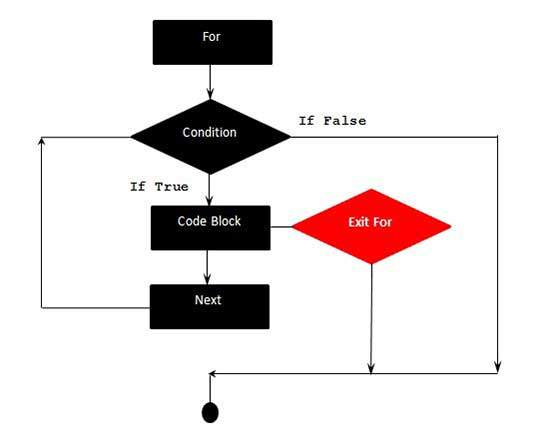# VBA - Exit For

A Exit For statement is used when we want to exit the For Loop based on certain criteria. When Exit For is executed, the control jumps to the next statement immediately after the For Loop.

## Syntax

Following is the syntax for Exit For Statement in VBA.

``` Exit For
```

## Flow Diagram### Example

The following example uses Exit For. If the value of the Counter reaches 4, the For Loop is exited and the control jumps to the next statement immediately after the For Loop.

```Private Sub Constant_demo_Click()
Dim a As Integer
a = 10

For i = 0 To a Step 2 'i is the counter variable and it is incremented by 2
MsgBox ("The value is i is : " & i)
If i = 4 Then
i = i * 10 'This is executed only if i=4
MsgBox ("The value is i is : " & i)
Exit For 'Exited when i=4
End If
Next
End Sub
```

When the above code is executed, it prints the following output in a message Box.

```The value is i is : 0

The value is i is : 2

The value is i is : 4

The value is i is : 40
```
vba_loops.htm